## Total Pressure Family of Inlet Boundary Conditions

Total pressure family of inlet boundary conditions prescribe total pressure at the inlet. Total pressure can be viewed as a Head of the turbines or a transport height of pumps. The benefit of keeping constant total pressure is you prescribe and keep constant the pressure drop (head, transport height). NOTE: For incompressible fluid flow, all the pressure dimensions are always [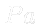/{rho}]=[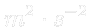], sometimes called kinematic pressure. So, all the pressures are divided by the density. Also all the mass flow rates, torques and powers are like divided by density. For incompressible fluid flow, the pressure value is alway relative, not absolute (this is why the zero value is often prescribed at the outlet). For example if we want to simulate the Total Head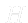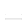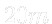, the total pressure would be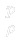=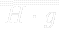=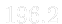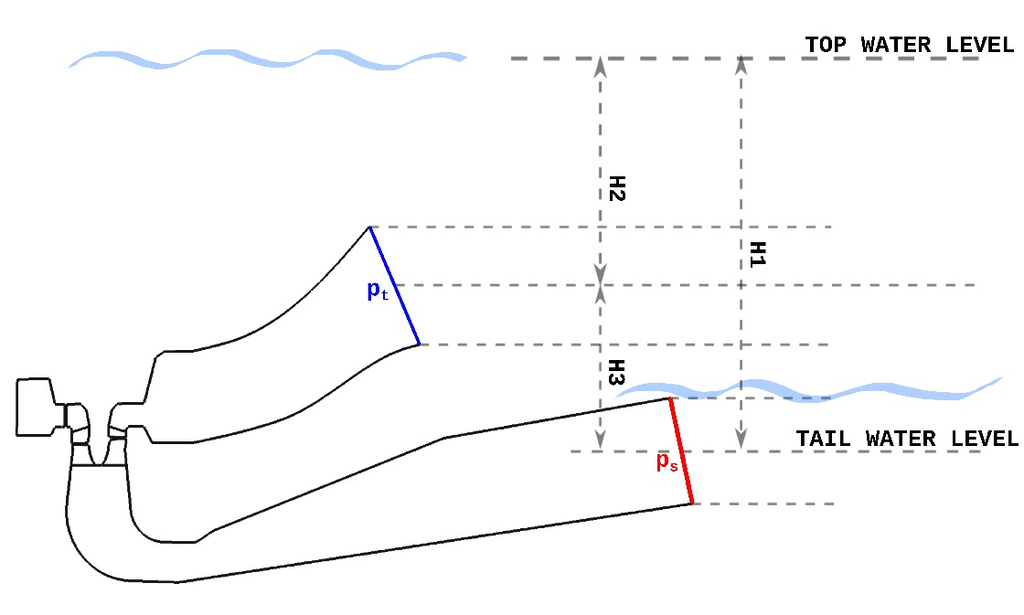Figure: General water machine. Sketch of water levels for boundary conditions.